## Thursday, 7 August 2014

### chapter-12 -worked-out-examples-5

 Example: 9
For two events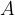$A$ and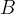$B$, we are given the following information: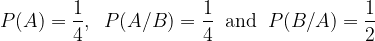$P(A) = \dfrac{1}{4},\;\;P(A/B) = \dfrac{1}{4}\;\;{\rm{and}}\;\;P(B/A) = \dfrac{1}{2}$
(a) Determine whether the two events are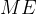$ME$ or independent, or neither.
(b) Find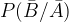$P(\bar B/\bar A)$. The notation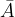$\bar A$ stands for the complementary event of event$A$, i.e.,$\bar A$ occurs if$A$ does not occur.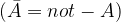$(\bar A = not - A)$
 Solution: 9-(a)
Since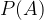$P(A)$ and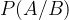$P(A/B)$ are the same, this means that$A$ and$B$ are independent events. Hence they are obviously not$ME$.
We therefore obtain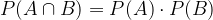$P(A \cap B) = P(A) \cdot P(B)$ (independence)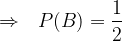$\Rightarrow \,\,\,\, P(B) = \dfrac{1}{2}$
 Solution: 9-(b)
Now, if$A$ and$B$ are independent events, then so are$\bar A$ and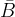$\bar B$ (and in fact ($A$ and$\bar B$) and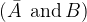$(\bar A\;\,{\rm{and}}\,B)$. This fact should be intuitively obvious but let us justify it with some rigor. We have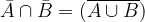$\bar A \cap \bar B = (\overline {A \cup B} )$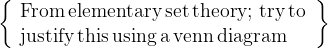$\left\{ \begin{array}{l} {\rm{From}}\,{\rm{elementary\, set \,theory; \,try\, to\, }}\\ {\rm{justify\, this\, using\, a \,venn\, diagram\,}} \end{array} \right\}$$\Rightarrow \,\,\,\, P(\bar A \cap \bar B) = P(\overline {A \cup B} )$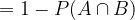$= 1 - P(A \cap B)$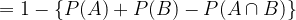$= 1 - \left\{ {P(A) + P(B) - P(A \cap B)} \right\}$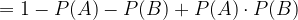$= 1 - P(A) - P(B) + P(A) \cdot P(B)$ { since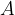$A$,$B$ are independent }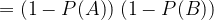$= (1 - P(A))\;(1 - P(B))$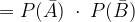$= P(\bar A)\; \cdot \;P(\bar B)$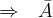$\Rightarrow\,\,\,\, \bar A$ and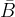$\bar B$ are independent
Similar proofs follow for the other two pairs.
Returning to the question, we see that since$\bar A$ and$\bar B$ are independent, we have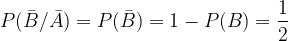$P(\bar B/\bar A) = P(\bar B) = 1 - P(B) = \dfrac{1}{2}$
 Example: 10
 In this example, we’ll get a taste of what is known as inverse probability, which we’ll of course be discussing in detail in a later section. A man is known to speak the truth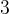$3$ out of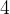$4$ times. He throws a die and tells you that he obtained a six. What is the chance of it being really a six ?
 Solution: 10
The problem here is that some event has already taken place, i.e., the throwing of a die. What we are required to do is find out how truthful the result being told to us is, given that the person reporting the result is not exactly a saint!
So, we first let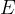$E$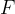$F$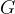$G$ denote the following events:$E$ : Man reports a six$F$ : Die shows a six$G$ : Die does not show a six
Observe that we are required to find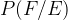$P(F/E)$, i.e., the probability of the die actually showing a six given that the man is reporting so.
Since we already know a bit about conditional probabilities, let us try to follow that route of manipulation.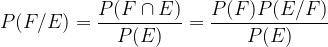$P(F/E) = \dfrac{{P(F \cap E)}}{{P(E)}} = \dfrac{{P(F)P(E/F)}}{{P(E)}}$
Now,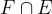$F \cap E$ is the event that the man reports a six when actually he obtains a six. The probability of this event will simply be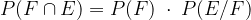$P(F \cap E) = P(F)\; \cdot \;P(E/F)$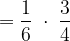$= \dfrac{1}{6}\; \cdot \;\dfrac{3}{4}$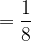$= \dfrac{1}{8}$
You are urged to carefully understand how we arrived at this.
Next, we try to find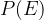$P(E)$, i.e., the probability of the man reporting a six. This can happen in two mutually exclusive ways.
 Way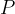$P$(Way) Actual result : Six : Man says the truth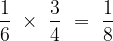$\dfrac{1}{6}\,\, \times \,\,\dfrac{3}{4}\,\, = \,\,\dfrac{1}{8}$ Actual result : Not a six : Man lies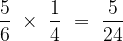$\dfrac{5}{6}\,\, \times \,\,\dfrac{1}{4}\,\, = \,\,\dfrac{5}{{24}}$
Thus,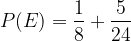$P(E) = \dfrac{1}{8} + \dfrac{5}{{24}}$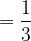$= \dfrac{1}{3}$
Finally,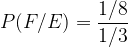$P(F/E) = \dfrac{{1/8}}{{1/3}}$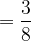$= \dfrac{3}{8}$
It should be remarked that a lot of readers might not understand fully the underlying idea of this problem. They need not despair since inverse probabilities will be dealt with in much detail later.